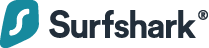# How to Convert Seconds to Hours and Minutes in JavaScript

To convert seconds to hours and minutes in JavaScript:

1. Divide the seconds by 60 to get the total minutes. The remainder will be the seconds in the output.
2. Divide the total minutes by 60 to get the hours. The whole number in the result will be the hours, and the remainder will be the minutes in the output.

For example:

``````function toHoursAndMinutes(totalSeconds) {
const totalMinutes = Math.floor(totalSeconds / 60);

const seconds = totalSeconds % 60;
const hours = Math.floor(totalMinutes / 60);
const minutes = totalMinutes % 60;

return { h: hours, m: minutes, s: seconds };
}

// { h: 0, m: 1, s: 0 }
console.log(toHoursAndMinutes(60));

// { h: 0, m: 16, s: 40 }
console.log(toHoursAndMinutes(1000));

// { h: 1, m: 10, s: 50 }
console.log(toHoursAndMinutes(4250));
``````

We create a reusable function that takes a total number of seconds and returns an object containing the separate hours, minutes, and seconds values.

First, we divide the total seconds by 60 to get the number of total minutes.

``````console.log(60 / 60); // 1

console.log(1000 / 60); // 16.666666666666668

console.log(4250 / 60); // 70.83333333333333
``````

The division results in a decimal number, so we use the `Math.floor()` function to get just the whole number of the result.

`Math.floor()` returns the largest integer less than or equal to a specified number.

``````console.log(Math.floor(10.95)); // 10
console.log(Math.floor(10)); // 10
console.log(Math.floor(10.05)); // 10``````

After this, we use the modulo operator (`%`) to get the division’s remainder for the seconds in the result.

``````console.log(60 % 60); // 0

console.log(1000 % 60); // 40

console.log(4250 % 60); // 50
``````

Tip: Skip this step if the seconds should not be in the result.

We all know 60 seconds make a minute, and 60 minutes make an hour. After getting the total minutes, we do something similar to what we just did to the total seconds value: we divide the total minutes by 60 to get the hours and use the module operator to get the division’s remainder, which will be the minutes in the output.

After getting all the values, we return an object with `h`, `m`, and `s` properties containing the hours, minutes, and seconds respectively.

``return { h: hours, m: minutes, s: seconds };``

### Surfshark VPN: Your ultimate cyber shieldProtecting your connection is key. Surfing the web, you're exposed to many risks: data breaches, hacking attacks, & snoopers. One solution to fight them all — a VPN.

## Convert seconds to `HH:mm:ss`

Instead of an object, you might want the result to be a time string in a format like `HH:mm:ss`, where each value is separated by a colon and has a minimum of two digits in the string. Here’s how we can produce such a time string.

``````function toTimeString(totalSeconds) {
const totalMs = totalSeconds * 1000;
const result = new Date(totalMs).toISOString().slice(11, 19);

return result;
}

console.log(toTimeString(60)); // 00:01:00

console.log(toTimeString(1000)); // 00:16:40

console.log(toTimeString(4250)); // 01:10:50
``````

First, we convert the seconds to milliseconds to pass it to the `Date()` constructor and create a new `Date` object.

The `Date` `toISOString()` method returns a string representation of the date in the ISO 8601 format, i.e., `YYYY-MM-DDTHH:mm:ss.sssZ`

``````const totalMs = 60000;

// 1970-01-01T00:01:00.000Z
console.log(new Date(totalMs).toISOString());``````

You can see that `toISOString()` does the conversion for us. We just need a way to extract the `HH:mm:ss` from the ISO string.

The `String slice()` method returns the portion of a string between specified start and end indexes, passed as the first and second arguments respectively.

The `HH:mm:ss` starts at index 11 of the string and ends at index 18. So to extract it, the values we pass to `slice()` or `substring()` will be `11` and `19`.

Tip: `19` because `slice()` excludes the character at the end index from the resulting substring.

``````// 00:01:00
console.log('1970-01-01T00:01:00.000Z'.slice(11, 19));
``````

To exclude the seconds from the result, we just need to reduce the end index by 3 to exclude the colon and the digits of the seconds.

``````// 00:01
console.log('1970-01-01T00:01:00.000Z'.slice(11, 16));
``````

## 11 Amazing New JavaScript Features in ES13

This guide will bring you up to speed with all the latest features added in ECMAScript 13. These powerful new features will modernize your JavaScript with shorter and more expressive code.1.